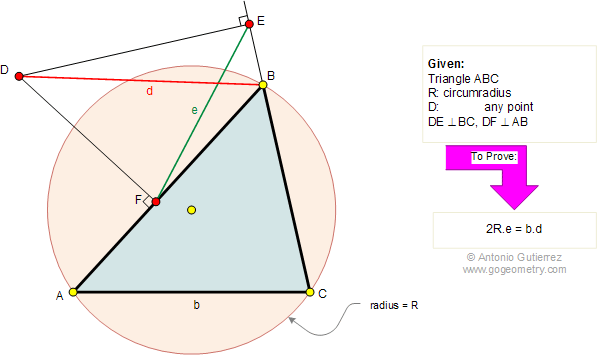# Geometry Problem 291: Triangle, Circle, Circumradius, Perpendicular.

### Proposition

In the figure below, ABC is a triangle with circumradius R. DE is perpendicular to BC and DF is perpendicular to AB. If AC = b, BD = d, and EF =e, prove that 2R.e = b.d.